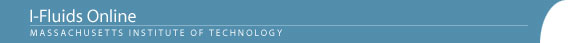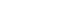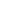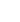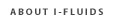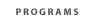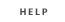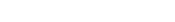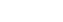2.25: Advanced Fluid Dynamics Section 1: Continuum viewpoint and the equation of motion Section 2: Static Fluids Section 3: Mass Conservation Section 4: Inviscid flow - differential approach Section 5: Control Volume Theorums Section 6: Navier-Stokes equation and viscous flow Section 7: Similarity and dimensional analysis Section 8: Boundary layers, separation and effect on drag/lift Section 9: Vorticity and circulation Section 10: Potential flows; lift, drag and thrust production Section 11: Surface tension and its effect on flows Section 12: Introduction to turbulence Back to 2.25 HomeSection 6: Viscous Flows6.1 The equation of motion for viscous flows. Surface stress; stress tensor; symmetry of the stress tensor; the equation of motion in terms of the stress tensor; the stress tensor for Newtonian fluids; the Navier-Stokes equations; summary of the governing equations and boundary conditions for incompressible flows and constant-density flows; boundary conditions for viscous flows.
6.2 Comments on the character of the Navier-Stokes equations at low and high Reynolds numbers; laminar flows and their stability; turbulence.
6.3 Some truly inertia-free flows: Steady, laminar fully developed pipe flows; laminar Couette flows with and without pressure gradient.
6.4 (Almost) inertia-free flows. Criteria for quasi-steady, locally-fully-developed (quasi-parallel) laminar flow. Examples: Flows in various converging and diverging channels, free-surface flows, and lubrication theory.
6.5 Rayleigh's problem of the transient motion induced by a flat plate that moves in its own plane: an archetypal example of laminar viscous flow with significant inertial effects. The viscous diffusion time and its implications in various types of flows, including boundary layers in steady laminar flow.

Reading

Special 2.25 notes by A. Sonin: (i) Equation of Motion for Viscous Fluids [PDF] and (ii) Criteria for inertia-free and locally fully-developed flows

Fay, Chapter 6

Problem Set Section 6

Problem 6.3
Problem 6.6
Problem 6.10
Problem 6.13
Problem 6.16
Problem 6.20
Problem 6.22
Problem 8.3